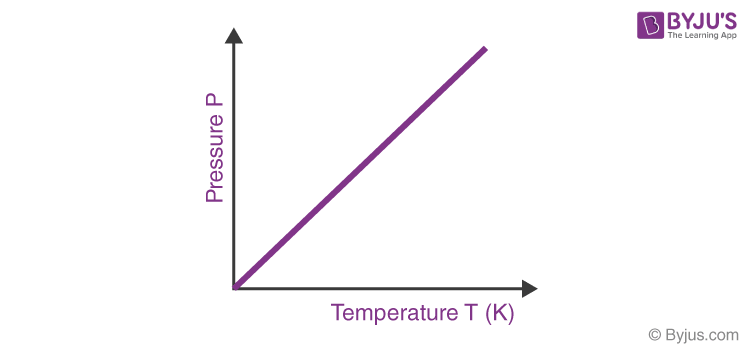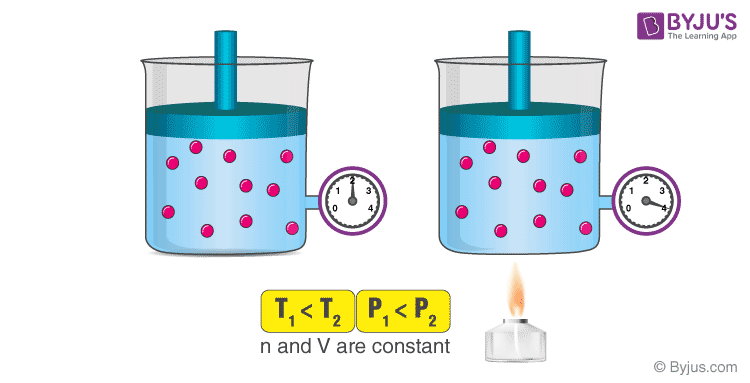# Gay-Lussac’s Law

## What is Gay-Lussac’s Law?

Gay-Lussac’s law is a gas law which states that the pressure exerted by a gas (of a given mass and kept at a constant volume) varies directly with the absolute temperature of the gas. In other words, the pressure exerted by a gas is proportional to the temperature of the gas when the mass is fixed and the volume is constant.

This law was formulated by the French chemist Joseph Gay-Lussac in the year 1808. The mathematical expression of Gay-Lussac’s law can be written as follows:

P ∝ T ; P/T = k

Where:

• P is the pressure exerted by the gas
• T is the absolute temperature of the gas
• k is a constant.

The relationship between the pressure and absolute temperature of a given mass of gas (at constant volume) can be illustrated graphically as follows.From the graph, it can be understood that the pressure of a gas (kept at constant volume) reduces constantly as it is cooled until the gas eventually undergoes condensation and becomes a liquid.

## Recommended Videos## Formula and Derivation

Gay-Lussac’s law implies that the ratio of the initial pressure and temperature is equal to the ratio of the final pressure and temperature for a gas of a fixed mass kept at a constant volume. This formula can be expressed as follows:

(P1/T1) = (P2/T2)

Where:

• P1 is the initial pressure
• T1 is the initial temperature
• P2 is the final pressure
• T2 is the final temperature

This expression can be derived from the pressure-temperature proportionality for gas. Since P ∝ T for gases of fixed mass kept at constant volume:

P1/T1 = k (initial pressure/ initial temperature = constant)

P2/T2 = k (final pressure/ final temperature = constant)

Therefore, P1/T1 = P2/T2 = k

Or, P1T2 = P2T1

## Examples of Gay-Lussac’s Law

When a pressurized aerosol can (such as a deodorant can or a spray-paint can) is heated, the resulting increase in the pressure exerted by the gases on the container (owing to Gay-Lussac’s law) can result in an explosion. This is the reason why many pressurized containers have warning labels stating that the container must be kept away from fire and stored in a cool environment.An illustration describing the increase in pressure which accompanies an increase in the absolute temperature of a gas kept at a constant volume is provided above. Another example of Gay-Lussac’s law can be observed in pressure cookers. When the cooker is heated, the pressure exerted by the steam inside the container increases. The high temperature and pressure inside the container cause the food to cook faster.## Solved Exercises on Gay-Lussac’s Law

### Exercise 1

The pressure of a gas in a cylinder when it is heated to a temperature of 250K is 1.5 atm. What was the initial temperature of the gas if its initial pressure was 1 atm.

Given,

Initial pressure, P1 = 1 atm

Final pressure, P2 = 1.5 atm

Final temperature, T2 = 250 K

As per Gay-Lussac’s Law, P1T2 = P2T1

Therefore, T1 = (P1T2)/P2 = (1*250)/(1.5) = 166.66 Kelvin.

### Exercise 2

At a temperature of 300 K, the pressure of the gas in a deodorant can is 3 atm. Calculate the pressure of the gas when it is heated to 900 K.

Initial pressure, P1 = 3 atm

Initial temperature, T1 = 300K

Final temperature, T2 = 900 K

Therefore, final pressure (P2) = (P1T2)/T1 = (3 atm*900K)/300K = 9 atm.

## Frequently Asked Questions – FAQs

### What is Gay Lussac’s law formula?

The law of Gay-Lussac is a variant of the ideal gas law where the volume of gas is held constant. The pressure of a gas is directly proportional to its temperature while the volume is kept constant. P / T = constant or Pi / Ti = Pf / Tf are the standard calculations for Gay-Lussac ‘s law.

### What does Charles law state?

The physical theory known as Charles’ law states that a gas’s volume equals a fixed value as determined on the Kelvin scale compounded by its temperature.

### What is the importance of Gay Lussac’s law?

The meaning of this gas law is that it illustrates that rising a gas’s temperature induces a relative increase in its pressure (assuming that the volume does not change). Likewise, reducing the temperature allows the strain to decrease proportionally.

### How does Avogadro’s law apply to everyday life?

The Law of Avogadro states that the amount of the gas is directly proportional to the number of gas moles. You are driving more molecules of gas into it when you blow up a football.

### What are the applications of Avogadro’s law?

The relationship between a gas’s relative vapour density and its relative molecular mass is defined. Establishes the relationship between the volume of a gas at STP and gram molecular weight.

Test your knowledge on Gay Lussacs Law

1. Angela chekwe

Good

2. Md moin pasha

Best explaination

3. Than you for a very clear explanation.

4. Clear explanation with short content.
Examples are the best way to understand the concept! I have understood the whole thing.

5. Kushagra

This is too good man
Thanks 🙏🙏🙏

6. Anuhya

Thank you for best and clear explanation. Thank you so much

7. This notes make my study more better

8. Thanks for ur explanation

9. ozlem tuna